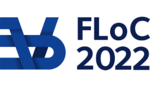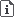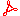FLOC 2022: FEDERATED LOGIC CONFERENCE 2022
IJCAR ON WEDNESDAY, AUGUST 10TH
Days:
previous day
all days

View: session overviewtalk overviewside by side with other conferences

08:30-09:00Coffee & Refreshments
09:00-10:30 Session 110D: System Evolution and Termination
09:00
CTL* model checking for data-aware dynamic systems with arithmeticPRESENTER: Sarah Winkler

ABSTRACT. The analysis of complex dynamic systems is a core research topic in formal methods and AI, and combined modelling of systems with data has gained increasing importance in applications such as business process management. In addition, process mining techniques are nowadays used to automatically mine process models from event data, often without correctness guarantees. Thus verification techniques for linear and branching time properties are needed to ensure desired behavior. Here we consider data-aware dynamic systems with arithmetic (DDSAs), which constitute a concise but expressive formalism of transition systems with linear arithmetic guards. We present a CTL* model checking procedure for DDSAs that relies on a finite-state abstraction by means of a set of formulas that capture variable configurations. Linear-time verification was shown to be decidable in specific classes of DDSAs where the constraint language or the control flow are suitably confined. We investigate several of these restrictions for the case of CTL*, with both positive and negative results: CTL* verification is proven decidable for monotonicity and integer periodicity constraint systems, but undecidable for feedback free and bounded lookback systems. To witness the feasibility of our approach, we implemented it in the SMT-based prototype ada. It turns out that many practical business process models can be effectively analyzed by our tool.

09:20
Implicit Definitions with Differential Equations for KeYmaera X (System Description)PRESENTER: Yong Kiam Tan

ABSTRACT. Definition packages in theorem provers provide users with means of defining and organizing concepts of interest. This system description presents a new definition package for the hybrid systems theorem prover KeYmaera X based on differential dynamic logic (dL). The package adds KeYmaera X support for user-defined smooth functions whose graphs can be implicitly characterized by dL formulas. Notably, this makes it possible to implicitly characterize functions, such as the exponential and trigonometric functions, as solutions of differential equations and then prove properties of those functions using dL's differential equation reasoning principles. Trustworthiness of the package is achieved by minimally extending KeYmaera X's soundness-critical kernel with a single axiom scheme that expands function occurrences with their implicit characterization. Users are provided with a high-level interface for defining functions and non-soundness-critical tactics that automate low-level reasoning over implicit characterizations in hybrid system proofs.

09:35
On eventual non-negativity and positivity for the weighted sum of powers of matricesABSTRACT. The long run behaviour of linear dynamical systems is often studied by looking at eventual properties of matrices and recurrences that underlie the system. A basic problem that lies at the core of many questions in this setting is the following: given a set of pairs of rational weights and matrices {(w1, A1), . . . , (wm, Am)}, we ask if the weighted sum of powers of these matrices is eventually non-negative (resp. positive), i.e., does there exist an integer N s.t for all n greater than N , (w1.A1^n + w2.A2^n+ ... + w_m.Am^n) \geq 0 (resp. > 0). The restricted setting when m = w1 = 1, results in so-called eventually non-negative (or eventually positive) matrices, which enjoy nice spectral properties and have been well-studied in control theory. More applications arise in varied contexts, ranging from program verification to partially observable and multi-modal systems.

Our goal is to investigate this problem and its link to linear recurrence sequences. Our first result is that for m > 1, the problem is as hard as the ultimate positivity of linear recurrences, a long standing open question (known to be coNP-hard). Our second result is a reduction in the other direction showing that for any m \geq 1, the problem reduces to ultimate positivity of linear recurrences. This shows precise upper bounds for several subclasses of matrices by exploiting known results on linear recurrence sequences. Our third main result is an effective algorithm for the class of diagonalizable matrices, which goes beyond what is known on linear recurrence sequences.

09:55
Proving Non-Termination and Lower Runtime Bounds with LoAT (System Description)PRESENTER: Florian Frohn

ABSTRACT. We present the Loop Acceleration Tool (LoAT), a powerful tool for proving non-termination and worst-case lower bounds for programs operating on integers. It is based on a novel calculus for loop acceleration, i.e., transforming loops into non-deterministic straight-line code, and for finding non-terminating configurations. To implement it efficiently, LoAT uses a new approach based on unsat cores. We evaluate LoAT's power and performance by extensive experiments.

10:10
Automatic Complexity Analysis of Integer Programs via Triangular Weakly Non-Linear LoopsPRESENTER: Nils Lommen

ABSTRACT. There exist several results on deciding termination and computing runtime bounds for triangular weakly non-linear loops (twn-loops). We show how to use such results on subclasses of programs where complexity bounds are computable within incomplete approaches for complexity analysis of full integer programs. To this end, we present a novel modular approach which computes local runtime bounds for subprograms which can be transformed into twn-loops. These local runtime bounds are then lifted to global runtime bounds for the whole program. The power of our approach is shown by our implementation in the tool KoAT which analyzes complexity of programs where all other state-of-the-art tools fail.

10:30-11:00Coffee Break
11:00-12:30 Session 112D: Decidable Logics and Models
11:00
PRESENTER: Sophie Tourret

ABSTRACT. Abduction is the task of finding possible extensions of a given knowledge base that would make a given sentence logically entailed. As such, it can be used to explain why the sentence does not follow, to repair incomplete knowledge bases, and to provide possible explanations for unexpected observations. We consider TBox abduction in the lightweight description logic EL, where the observation is a concept inclusion and the background knowledge is a description logic TBox. To avoid useless answers, such problems usually come with further restrictions on the solution space and/or minimality criteria that help sort the chaff from the grain. We argue that existing minimality notions are insufficient, and introduce connection minimality. This criterion rejects hypotheses that use concept inclusions “disconnected” from the problem at hand. We show how to compute a special class of connection-minimal hypotheses in a sound and complete way. Our technique is based on a translation to first-order logic, and constructs hypotheses based on prime implicates. We evaluate a prototype implementation of our approach on ontologies from the medical domain.

11:20
PRESENTER: Lucas Bueri

ABSTRACT. We consider a logic used to describe sets of configurations of distributed systems, whose network topologies can be changed at runtime, by reconfiguration programs. The logic uses inductive definitions to describe networks with an unbounded number of components and interactions, written using a multiplicative conjunction, reminiscent of Bunched Implications and Separation Logic. We study the complexity of the satisfiability and entailment problems for the configuration logic under consideration. Additionally, we consider degree boundedness (is every component involved in a bounded number of interactions?), an ingredient for decidability of entailments.

11:40
PRESENTER: Clark Barrett

ABSTRACT. Dynamic arrays, also referred to as vectors, are fundamental data structures used in many programs. Modeling their semantics efficiently is crucial when reasoning about such programs. The theory of arrays is widely supported but is not ideal, because the number of elements is fixed (determined by its index sort) and cannot be adjusted, which is a problem, given that the length of vectors often plays an important role when reasoning about vector programs. In this paper, we propose reasoning about vectors using a theory of sequences. We introduce the theory, propose a basic calculus adapted from one for the theory of strings, and extend it to efficiently handle common vector operations. We prove that our calculus is sound and show how to construct a model when it terminates with a saturated configuration. Finally, we describe an implementation of the calculus in cvc5 and demonstrate its efficacy by evaluating it on verification conditions for smart contracts and benchmarks derived from existing array benchmarks.

12:00
Flexible Proof Production in an Industrial-Strength SMT SolverPRESENTER: Haniel Barbosa

ABSTRACT. Proof production for SMT solvers is paramount to ensure their correctness independently from implementations, which are often prohibitively complex to be verified. However, historically SMT proof production has struggled with performance and coverage issues, with many crucial solving techniques being disabled, and lack of details, with hard to check, coarse-grained proofs. Here we present a flexible proof production architecture designed to handle the complexity of versatile, industrial-strength SMT solvers, and how we leveraged it to produce detailed proofs, including for previously unsupported components by any solver. The architecture allows proofs to be produced modularly, lazily and with numerous safeguards for correct generation. This architecture has been implemented in the state-of-the-art SMT solver cvc5. We evaluate its proofs for SMT-LIB benchmarks and show the new architecture allows better coverage than previous approaches, has acceptable performance overhead, and allows detailed proofs for most solving components.

12:30-14:00Lunch Break

Lunches will be held in Taub lobby (CAV, CSF) and in The Grand Water Research Institute (DL, IJCAR, ITP).

14:00-15:30 Session 115C: Invited Talk and Optimized Reasoning
14:00
Using Automated Reasoning Techniques for Enhancing the Efficiency and Security of (Ethereum) Smart ContractsPRESENTER: Elvira Albert
15:00

ABSTRACT. Automated theorem provers (ATPs) are today used to attack open problems in several areas of mathematics. An ongoing project by Kinyon and Veroff uses Prover9 to search for the proof of the Abelian Inner Mapping (AIM) Conjecture, one of the top open conjectures in quasigroup theory. In this work, we improve Prover9 on a benchmark of AIM problems by neural synthesis of useful alternative formulations of the goal. In particular, we design the 3SIL (stratified shortest solution imitation learning) method. 3SIL trains a neural predictor through a reinforcement learning (RL) loop to propose correct rewrites of the conjecture that guide the search. 3SIL is first developed on a simpler, Robinson arithmetic rewriting task for which the reward structure is similar to theorem proving. There we show that 3SIL outperforms other RL methods. Next we train 3SIL on the AIM benchmark and show that the final trained network, deciding what actions to take within the equational rewriting environment, proves 70.2% of problems, outperforming Waldmeister (65.5%). When we combine the rewrites suggested by the network with Prover9, we prove 8.3% more theorems than Prover9 in the same time, bringing the performance of the combined system to 90%

15:30-16:00Coffee Break
16:00-17:00 Session 116C: Modalities and Decidability
16:00
PRESENTER: Stepan Kuznetsov

ABSTRACT. Adding multi-modalities (called subexponentials) to linear logic enhances its power as a logical framework, which has been extensively used in the specification of e.g. proof systems, programming languages and bigraphs. Initially, subexponentials allowed for classical, linear, affine or relevant behaviors. Recently, this framework was enhanced so to allow for commutativity as well. In this work, we close the cycle by considering associativity. We show that the resulting system (acLL) admits the (multi)cut rule, and we prove two undecidability results for fragments/variations of acLL.

16:20

ABSTRACT. We introduce a~paraconsistent modal logic $\KGsquare$, based on Gödel logic with coimplication (bi-Gödel logic) expanded with a De Morgan negation $\neg$. We use the logic to formalise reasoning with graded, incomplete and inconsistent information. Semantics of $\KGsquare$ is two-dimensional: we interpret $\KGsquare$ on crisp frames with two valuations $v_1$ and $v_2$, connected via $\neg$, that assign to each formula two values from the real-valued interval $[0,1]$. The first (resp., second) valuation encodes the positive (resp., negative) information the state gives to a~statement. We obtain that $\KGsquare$ is strictly more expressive than the classical modal logic $\mathbf{K}$ by proving that finitely branching frames are definable and by establishing a faithful embedding of $\mathbf{K}$ into $\KGsquare$. We also construct a~constraint tableau calculus for $\KGsquare$ over finitely branching frames, establish its decidability and provide a~complexity evaluation.

16:40
PRESENTER: Yoni Zohar

ABSTRACT. A four-valued semantics for the modal logic K is introduced. Possible worlds are replaced by a hierarchy of four-valued valuations, where the valuations of the first level correspond to valuations that are legal w.r.t. a basic non-deterministic matrix, and each level further restricts its set of valuations. The semantics is proven to be effective, and to precisely capture derivations in a sequent calculus for K of a certain form. Similar results are then obtained for the modal logic KT, by simply deleting one of the truth values.